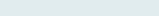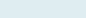# Count Days from Specific Date

Suppose you want to count the number of working days along with text and show day names in Excel. It gives the number of days on the basis present date to the given date after excluding weekends (default) with text in the same cell.

Query: We have dates. Our task is to get the number of working days from today’s date ignoring weekends.

Formula to implement:

=NETWORKDAYS(B5,TODAY()) & ” No leave”

Function applicable:

Networkdays: The Excel NETWORKDAYS function (net workdays) returns a number of working days between two given dates.

## Parameters

start_date – First date or string of a date.
end_date – Second date or string of a date
holidays [optional] – A list of additional days to exclude apart from weekends.

Today: The Excel TODAY function returns the current date in the selected cell. Example; to show the current date in Excel automatically, use TODAY(), empty parenthesis.

Parameters

(Empty Parenthesis)

Formula explanation step by step

Use shortcut F9 function key in Excel, tab clicks on evaluating the formula.

Let’s break the formula into multiple small steps to understand its application.

First formula:

Step 1: TODAY ()

Result:  43874

it will return the current date.

Second formula: =NETWORKDAYS(B5,TODAY()) & ” No leave”

Syntax: NETWORKDAYS (start_date, end_date, [holidays])

Step 2:Result: 290 No leave
It will give the count of the number of days worked from the current date with text (No leave) in the same cell.

Note: “&” operator in Excel is a type of concatenate which is used to join two strings, values or results.

## Get Days Name from a given Date

Query: In case of user want to know the day name from a specific date, to get we use to choose and weekday function. Now you can get days name from a given date.

Formula to implement:

=CHOOSE(WEEKDAY(B5),”MON”,”TUE”,”WED”,”THU”,”FRI”,”SAT”,”SUN”)

Functions applicable:

Choose: The Excel CHOOSE function returns a specific cell information from the list of data points based on the given arguments.

Weekday: The Excel WEEKDAY function returns an integer between 1 and 7 the corresponds specific day of a week.

Formula explanation step by step

Let’s break the formula into multiple small steps to understand its application.

First formula:

Syntax: WEEKDAY(serial_number,[return_type])

Step1:Result: 05-01-2019

From the first formula it returns date in cell B5 (05-01-2019).

Second formula: =CHOOSE(WEEKDAY(B5),”SUN”,”MON”,”TUE”,”WED”,”THU”,”FRI”,”SAT”)

Syntax: =CHOOSE(index_num, value1, [value2], …)

Step 2: =CHOOSE(WEEKDAY(B5),”SUN”,”MON”,”TUE”,”WED”,”THU”,”FRI”,”SAT”)

Result: SAT

We get index number 7 from the given date. So, it will choose “SAT” as it is on seventh position in applied formula.

Second Option: You can also use text function =TEXT(B5,”dddd”) to get the day name “Saturday” from the given date in the cell (B5).